# Methods and formulas for the diagnostic measures in Analyze Binary Response for Definitive Screening Design

## Pearson residuals

Elements of the Pearson chi-square that can be used to detect ill-fitted factor/covariate patterns. Minitab stores the Pearson residual for the ith factor/covariate pattern. The formula is:

### Notation

TermDescription
yithe response value for the ith factor/covariate patternthe fitted value for the ith factor/covariate pattern
Vthe variance function for the model atThe following equation gives the variance function for a binomial model:

## Standardized and deleted Pearson residuals

Used to detect ill-fitted factor/covariate patterns. Minitab stores the standardized Pearson residual for the ith factor/covariate pattern. Deleted Pearson residuals are also called likelihood ratio Pearson residuals. For the deleted Pearson residual, Minitab calculates the one-step approximation described in Pregibon.1 This approximation is equal to the standardized Pearson residual. The formula is:

### Notation

TermDescription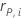the Pearson residual for the ith factor/covariate pattern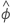1, for binomial models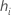the leverage for the ith factor/covariate pattern

## Deviance residuals

Deviance residuals are based on the model deviance and are useful in identifying ill-fitted factor/covariate patterns. The model deviance is a goodness-of-fit statistic based on the log-likelihood function. The deviance residual defined for the ith factor/covariate pattern is:

### Notation

TermDescription
yi the response value for the ith factor/covariate patternthe fitted value for the ith factor/covariate pattern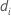the deviance for the ith factor/covariate pattern

## Standardized deviance residual

The standardized deviance residual is helpful in the identification of outliers. The formula is:

### Notation

TermDescription
rD,iThe deviance residual for the ith factor/covariate pattern
hiThe leverage for the ith factor/covariate pattern

## Deleted deviance residual

The deleted deviance residual measures the change in the deviance due to the omission of the ith case from the data. Deleted deviance residuals are also called likelihood ratio deviance residuals. For the deleted deviance residual, Minitab calculates a one-step approximation based on the Pregibon one-step approximation method1. The formula is as follows:

### Notation

TermDescription
yithe response value at the ith factor/covariate patternthe fitted value for the ith factor covariate pattern
hithe leverage for the ith factor/covariate pattern
r'D,ithe standardized deviance residual for the ith factor/covariate pattern
r'P,ithe standardized Pearson residual for the ith factor/covariate pattern

1. Pregibon, D. (1981). "Logistic Regression Diagnostics." The Annals of Statistics, Vol. 9, No. 4 pp. 705–724.

## Delta chi-square

Minitab calculates the change in the Pearson chi-square due to deleting all the observations with the jth factor/covariate pattern. Minitab stores one delta chi-square value for each distinct factor/covariate pattern in the data. You can use delta chi-square to detect ill-fitted factor/covariate patterns. The formula for the delta chi-square is:

### Notation

TermDescription
hj leverage
rj Pearson residuals

## Delta deviance

Minitab calculates the change in the deviance statistic by deleting all the observations with the jth factor/covariate pattern. Minitab stores one value for each distinct factor/covariate pattern in the data. You can use delta deviance to detect ill-fitted factor/covariate patterns. The change in the deviance statistic is:

### Notation

TermDescription
hjleverage
rjPearson residuals
djdeviance residuals

## Delta beta (standardized)

Minitab calculates the change by deleting all observations with the jth factor/covariate pattern. One value is stored for each distinct factor/covariate pattern in the data. You can use standardized delta β to detect factor/covariate patterns that have a strong influence on the estimates of the coefficients. This value is based on the standardized Pearson residual.

### Notation

TermDescription
hj leverage
rs j standardized Pearson residuals

## Delta beta

Minitab calculates the change by deleting all observations with the jth factor/covariate pattern. One value is stored for each distinct factor/covariate pattern in the data. You can use delta β to detect factor/covariate patterns that have a strong influence on the estimates of the coefficients. This value is based on the Pearson residual.

### Notation

TermDescription
hj leverage
rj Pearson residuals

## Leverages

The leverages are the diagonal elements of the generalized hat matrix. The leverages are useful in detecting factor/covariate patterns that may have a significant influence on the results.

### Notation

TermDescription
wj the jth diagonal element of the weight matrix from fitting the coefficients
xjthe jth row of the design matrix
Xthe design matrix
X'the transpose of X
Wthe weight matrix from the estimation of the coefficients

## Cook's distance

Minitab calculates an approximate Cook's distance.

### Notation

TermDescription
hithe leverage for the ith factor/covariate patternthe standardized Pearson residual for the ith factor/covariate pattern
pthe regression degrees of freedom

## DFITS

A measure of the influence of a single deletion on the fitted values. Observations with large DFITS values may be outliers. Minitab calculates an approximate value for DFITS.

### Notation

TermDescription
hiThe leverage for the data pointThe deleted Pearson residual for the data point

## Variance inflation factor (VIF)

To calculate a VIF, perform a weighted regression on the predictor with the remaining predictors. The weight matrix is that given in McCullagh and Nelder1 for the estimation of the coefficients. In this case, the VIF formula is equivalent to the formula for a linear regression. For example, for predictor xj the formula for the VIF is:

### Notation

TermDescription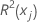coefficient of determination with xj as the response variable and the other terms in the model as the predictors

1. P. McCullagh and J. A. Nelder (1989). Generalized Linear Models, 2nd Edition, Chapman & Hall/CRC, London.

By using this site you agree to the use of cookies for analytics and personalized content.  Read our policy